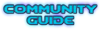## [Not a bug]Color averaging

Bugs that have been investigated and resolved somehow.

Moderator: GZDoom Developers

Forum rules

### Color averaging

Well, I don't know if this is a bug, but I'm working on averaging colors in Relighting using a different method described here.

I initially used the simple averging rather that the square root of average square as described for performance reasons, so I'm returning to that now that most performance issues are resolved. I got curious about GZDoom (really to see if an averaging function was already exposed) and see this in colormaps.cpp:

Code: Select all
`   // To calculate the blend it will just average the colors of the first map   if (fakecmaps.Size() > 1)   {      uint8_t map;      for (unsigned j = 1; j < fakecmaps.Size(); j++)      {         if (fileSystem.FileLength (fakecmaps[j].lump) >= 256)         {            int k, r, g, b;            auto lump = fileSystem.OpenFileReader (fakecmaps[j].lump);            lump.Read(map, 256);            r = g = b = 0;            for (k = 0; k < 256; k++)            {               r += GPalette.BaseColors[map[k]].r;               g += GPalette.BaseColors[map[k]].g;               b += GPalette.BaseColors[map[k]].b;            }            r /= 256;            g /= 256;            b /= 256;            // The calculated average is too dark so brighten it according to the palettes's overall brightness            int maxcol = max<int>(max<int>(palette_brightness, r), max<int>(g, b));                        fakecmaps[j].blend = PalEntry (255, r * 255 / maxcol, g * 255 / maxcol, b * 255 / maxcol);         }      }   }`

I've looked around a bit more, and I don't know if the above would be useful.
Hey Doomer

Joined: 25 Sep 2021

### Re: Color averaging

That function is for a very limited special case, so no, it won't be changed.Graf Zahl